## A ray of light (f = 5.09 x 1014 Hz) is incident on the boundary between air and an unknown material X at an angle of incidence of 550, as sh

Question

A ray of light (f = 5.09 x 1014 Hz) is incident on the boundary between air and an unknown material X at an angle of incidence of 550, as shown below. The absolute index of refraction (n) of material X is 1.66
A)Using the given information, identify a substance of which material X may be composed.
B)Using the given information, determine the speed of this ray of light in material X.
C)Using the given information, calculate the angle of refraction of the ray of light in material X.

in progress 0
2 months 2021-07-30T23:19:04+00:00 1 Answers 0 views 0

A) Material X is flint glass

B) Speed of light in the X material is 1.807 x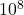m/s

C) Angle of refraction of light in medium X is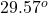Explanation:

Given data:

frequency of the light f = 5.09 x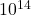Hz

angle of incidence Θ=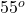index of refraction of material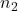= 1.66

A) To find material X

Given the index of refraction is 1.66 and hence the material is the flint glass

B) To calculate the speed of light in the material.

We know that the relation between index of refraction (n), velocity of light (c = 3 xm/s) and velocity of light is given by the equation:

n = c/v

Hence,

Speed of light in the X material v = c/n

=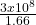= 1.807 xm/s

C) To calculate angle of refraction of light in medium X

We know that the Snell’s law states that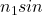Θ=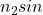Θ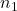= incident index= refracted index

Θ= incident angle

Θ= refracted angle

In given problem,= 1 since medium is air

Substituting the known values, we get

1 x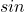= 1.66 xΘΘ=/1.66

= 0.4935

Hence, angle of refraction of light in medium X Θ=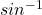(0.4935)

=